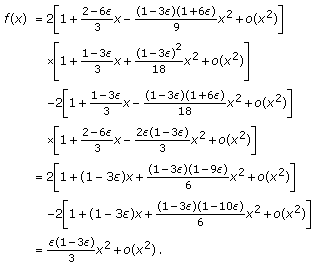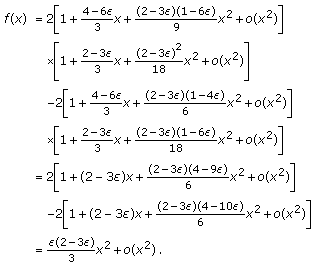# Two Sharp Inequalities for Power Mean, Geometric Mean, and Harmonic Mean

## Abstract

For, the power mean of orderof two positive numbersandis defined by. In this paper, we establish two sharp inequalities as follows:andfor all. Hereanddenote the geometric mean and harmonic mean ofandrespectively.

## 1. Introduction

For, the power mean of orderof two positive numbersandis defined by(11)

Recently, the power mean has been the subject of intensive research. In particular, many remarkable inequalities forcan be found in literature  . It is well known thatis continuous and increasing with respect tofor fixedand. If we denote byandthe arithmetic mean, geometric mean and harmonic mean ofand, respectively, then(12)

In , Alzer and Janous established the following sharp double-inequality (see also   [14,page 350]):(13)

for allIn , Mao proved(14)

for all, andis the best possible lower power mean bound for the sum.

The purpose of this paper is to answer the questions: what are the greatest valuesand, and the least valuesand, such thatandfor all?

## 2. Main Results

Theorem 2.1.for all, equality holds if and only if, andis the best possible lower power mean bound for the sum.

Proof.

If, then we clearly see that.

Ifand, then simple computation leads to(21)

Next, we prove thatis the best possible lower power mean bound for the sum.

For anyand, one has(22)

where.

Let, then the Taylor expansion leads to(23)

Equations (2.2) and (2.3) imply that for anythere exists, such thatfor.

Remark 2.2.

For any, one has(24)

Therefore,is the best possible upper power mean bound for the sum.

Theorem 2.3.for all, equality holds if and only if, andis the best possible lower power mean bound for the sum.

Proof.

If, then we clearly see thatIfand, then elementary calculation yields(25)

Next, we prove thatis the best possible lower power mean bound for the sum.

For anyand, one has(26)

where.

Let, then the Taylor expansion leads to(27)

Equations (2.6) and (2.7) imply that for anythere exists, such that(28)

for.

Remark 2.4.

For any, one has(29)

Therefore,is the best possible upper power mean bound for the sum.

## References

1. Wu SH: Generalization and sharpness of the power means inequality and their applications. Journal of Mathematical Analysis and Applications 2005,312(2):637–652. 10.1016/j.jmaa.2005.03.050

2. Richards KC: Sharp power mean bounds for the Gaussian hypergeometric function. Journal of Mathematical Analysis and Applications 2005,308(1):303–313. 10.1016/j.jmaa.2005.01.018

3. Wang WL, Wen JJ, Shi HN: Optimal inequalities involving power means. Acta Mathematica Sinica 2004,47(6):1053–1062.

4. Hästö PA: Optimal inequalities between Seiffert's mean and power means. Mathematical Inequalities & Applications 2004,7(1):47–53.

5. Alzer H, Qiu S-L: Inequalities for means in two variables. Archiv der Mathematik 2003,80(2):201–215. 10.1007/s00013-003-0456-2

6. Alzer H: A power mean inequality for the gamma function. Monatshefte für Mathematik 2000,131(3):179–188. 10.1007/s006050070007

7. Tarnavas CD, Tarnavas DD: An inequality for mixed power means. Mathematical Inequalities & Applications 1999,2(2):175–181.

8. Bukor J, Tóth J, Zsilinszky L: The logarithmic mean and the power mean of positive numbers. Octogon Mathematical Magazine 1994,2(1):19–24.

9. Pečarić JE: Generalization of the power means and their inequalities. Journal of Mathematical Analysis and Applications 1991,161(2):395–404. 10.1016/0022-247X(91)90339-2

10. Chen J, Hu B: The identric mean and the power mean inequalities of Ky Fan type. Facta Universitatis 1989, (4):15–18.

11. Imoru CO: The power mean and the logarithmic mean. International Journal of Mathematics and Mathematical Sciences 1982,5(2):337–343. 10.1155/S0161171282000313

12. Lin TP: The power mean and the logarithmic mean. The American Mathematical Monthly 1974, 81: 879–883. 10.2307/2319447

13. Alzer H, Janous W: Solution of problem 8*. Crux Mathematicorum 1987, 13: 173–178.

14. Bullen PS, Mitrinović DS, Vasić PM: Means and Their Inequalities, Mathematics and Its Applications (East European Series). Volume 31. D. Reidel, Dordrecht, The Netherlands; 1988:xx+459.

15. Mao QJ: Power mean, logarithmic mean and Heronian dual mean of two positive number. Journal of Suzhou College of Education 1999,16(1–2):82–85.

## Acknowledgments

This research is partly supported by N S Foundation of China under Grant 60850005 and the N S Foundation of Zhejiang Province under Grants Y7080185 and Y607128.

## Author information

Authors

### Corresponding author

Correspondence to Yu-Ming Chu.

## Rights and permissions

Reprints and Permissions

Chu, YM., Xia, WF. Two Sharp Inequalities for Power Mean, Geometric Mean, and Harmonic Mean. J Inequal Appl 2009, 741923 (2009). https://doi.org/10.1155/2009/741923

• Accepted:

• Published:

• DOI: https://doi.org/10.1155/2009/741923

### Keywords

• Simple Computation
• Taylor Expansion
• Elementary Calculation
• Calculation Yield
• Sharp Inequality